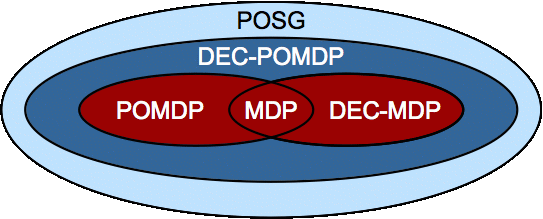## Dec-POMDP overview

As agents are built for ever more complex environments, methods that consider the uncertainty in the system have strong advantages. This uncertainty is common in domains such as autonomous navigation, inventory management,  sensor networks and e-commerce.  When choices are made in a decentralized manner by a set of decision makers, the problem can be modeled as a decentralized partially observable Markov decision process (Dec-POMDP).  While the Dec-POMDP model offers a rich framework for cooperative sequential decision making under uncertainty, the computational complexity of the model presents an important research challenge.  It is an extension of the partially observable Markov decision process (POMDP) framework and a specific case of a partially observable stochastic game (POSG) (see Hansen, et al., 2004).Dec-POMDPs represent a sequential problem. At each stage, each agent takes an action and receives:
• A local observation
• A joint immediate reward
A local policy for each agent is a mapping from its observation sequences to actions, Ω* → A (The state is unknown, so it may be beneficial to remember history)
A joint policy is a local policy for each agent
The goal is to maximize expected cumulative reward over a finite or infinite number of steps
Note that decisions for each agent are made solely on local information, but these decisions can affect the global reward and each agent's transitions and observations.

A Dec-POMDP can be described as follows:

M = <I, S, {Ai}, P, R, {Ωi}, O, h >

• I, the set of agents
• S, the set of states with initial state s0
• Ai, the set of actions for agent i, with A = ×iAi the set of joint actions
• P, the state transition probabilities: P(s'| s, a), the probability of the environment transitioning to state s' given it was in state s and agents took actions a
• R, the global reward function: R(s, a), the immediate reward the system receives for being in state s and agents taking actions a
• Ωi, the set of observations for agent i, with Ω = ×iΩi the set of joint observations
• O, the observation probabilities: O(o| s, a), the probability of agents seeing observations o, given the state is s and agents take actions a
• h, the horizon, whether infinite or if finite, a positive integer
• when h is infinite a discount factor, 0 ≤ γ < 1 , is used

A Dec-MDP is defined in the same way except:

• the state must be jointly fully observable. That is, the state is uniquely defined by the observations of all agents. So, if O(o| s, a) > 0 then P(s'| o)=1.

"... there is currently no good way to combine game theoretic and POMDP control strategies." -- Russell and Norvig, 2003

## Dec-POMDP algorithms

Optimal and approximate algorithms have been developed for general Dec-POMDPs as well as a number of subclasses. The worst case complexity of the general case has been shown to be NEXP-complete (Bernstein et al., 2002) with optimal solutions found by dynamic programming (Hansen et al., 2004) and latter by methods that can exploit structure when present. A reduction in complexity to NP is seen when the agents are mostly independent (Becker et al., 2003) and communication can be explicitly modeled and analyzed (Goldman et al., 2004).

For an overview of Dec-POMDPs, subclasses and algorithms, see our latest tutorial [pdf] [webpage].
Home